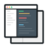# German Credit Dataset Analysis to Classify Loan Applications

##### In this data science project, you will work with German credit dataset using classification techniques like Decision Tree, Neural Networks etc to classify loan applications using R.#### Videos

Each project comes with 2-5 hours of micro-videos explaining the solution.#### Code & Dataset## What will you learn

Importing and understanding the dataset
Understanding unbalanced data and converting class into factors
Dividing the dataset into equal parts with equal distribution of both classes
Imputing for the null values
Defining cross-validation, metrics, and preprocessing techniques
Understanding and implementing LOGISTIC REGRESSION and selecting important features
Applying Bayesian model along with recursive partitioning
Improve Logistic Results using Random Forest
Defining AUC-ROC score and getting in-depth knowledge of how it works
Using Gini, AUC, and KS for evaluating model
Understanding Recall, Precision and F1score
Model Improvement with Gaussian RBF kernel
Display Performance Reports and interpreting the same
Visualizing the result of each model via plot

## Project Description

The German credit dataset contains information on 1000 loan applicants. Each applicant is described by a set of 20 different attributes. Of these 20 attributes, seventeen attributes are discrete while three are continuous. The main idea is to use techniques from the field of information theory to select a set of important attributes that can be used to classify tuples. In this data science project, you will train a neural network using these attributes; the neural network is then used to classify tuples.

## Similar Projects

#### Time Series Forecasting with LSTM Neural Network Python

Deep Learning Project- Learn to apply deep learning paradigm to forecast univariate time series data.

#### Data Science Project-TalkingData AdTracking Fraud Detection

Machine Learning Project in R-Detect fraudulent click traffic for mobile app ads using R data science programming language.

#### Forecast Inventory demand using historical sales data in R

In this machine learning project, you will develop a machine learning model to accurately forecast inventory demand based on historical sales data.

## Curriculum For This Mini Project

Introduction
07m
Import Data Sets
01m
Rename Columns
10m
Next Steps
00m
Import Libraries
06m
Distribution of Columns
04m
Different Approaches
02m
Weight of Evidence (WOE)
12m
Information Value (IV)
04m
Compute WOE and IV
06m
Univariate, Bivariate and Multivariate
06m
Logistic Regression Introduction
07m
Recap
01m
Library Overview
01m
Data Set Overview
02m
Next Steps - Overview
02m
Custom Functions
05m
Function to Compute WOE and IV
02m
Compute WOE and IV for each Variable
21m
Variable Clustering
05m
Training and Testing Sample
02m
Logistic Regression
11m
Logistic Regression - QnA
08m
Decision Tree
08m
Random Forest
04m
Logistic Regression using important variables
03m
Conditional Tree
01m
Bayesian Learn Model
03m
KSVM - Kernel Support Vector Machines
05m
Neural Network
04m
Compare all Models
05m